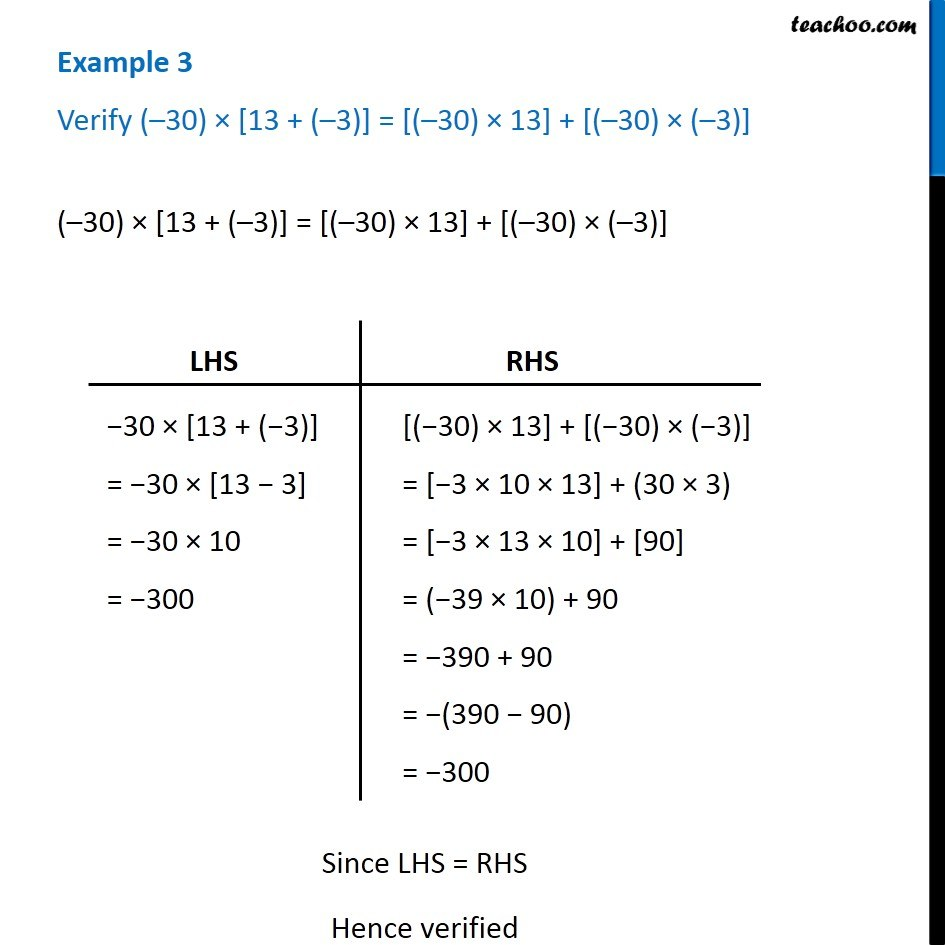Subscribe to our Youtube Channel - https://you.tube/teachoo

1. Chapter 1 Class 7 Integers
2. Concept wise
3. Distributive law in Integers

Transcript

Example 3 Verify ( 30) [13 + ( 3)] = [( 30) 13] + [( 30) ( 3)] ( 30) [13 + ( 3)] = [( 30) 13] + [( 30) ( 3)] LHS 30 [13 + ( 3)] = 30 [13 3] = 30 10 = 300 RHS [( 30) 13] + [( 30) ( 3)] = [ 3 10 13] + (30 3) = [ 3 13 10] +  = ( 39 10) + 90 = 390 + 90 = (390 90) = 300 Since LHS = RHS Hence verified

Distributive law in Integers